CBSE Class 10 Sample Paper for 2022 Boards - Maths Standard [Term 2]

Class 10
Solutions of Sample Papers for Class 10 Boards

## The internal and external radii of a spherical shell are 3cm and 5cm respectively. It is melted and recast into a solid cylinder of diameter 14cm, find the height of the cylinder. Also find the total surface area of the cylinder. ("Take 𝜋 = " 22/7)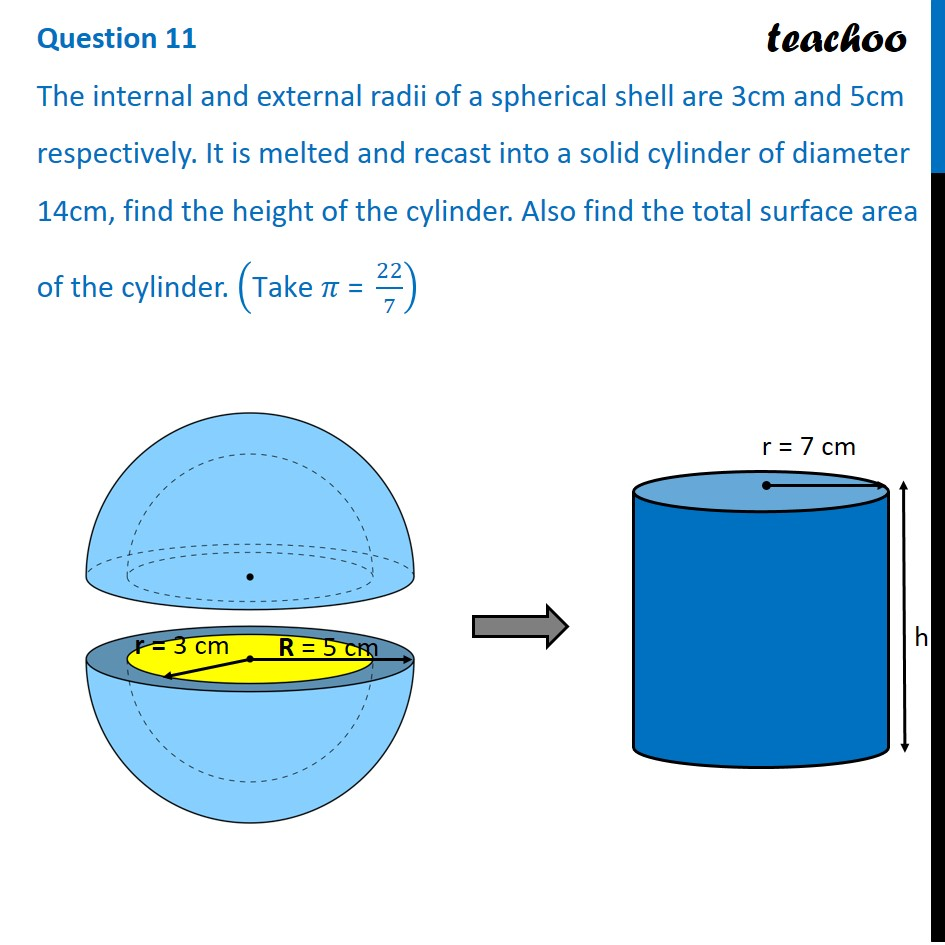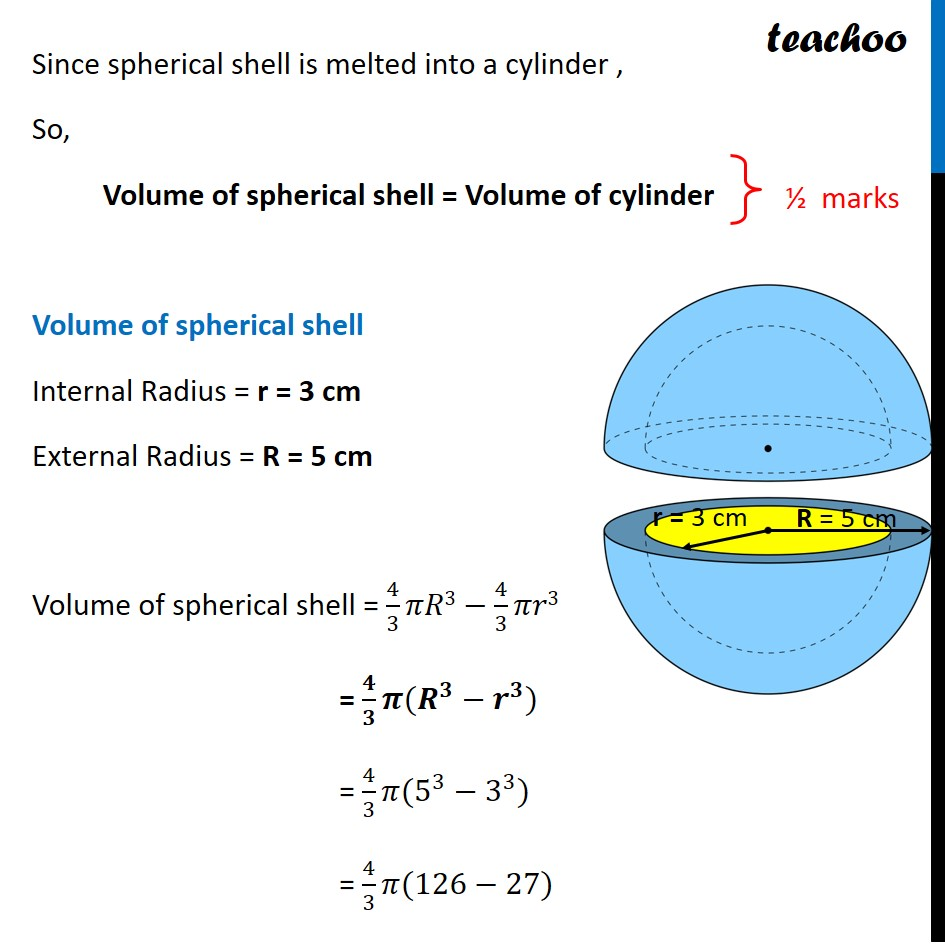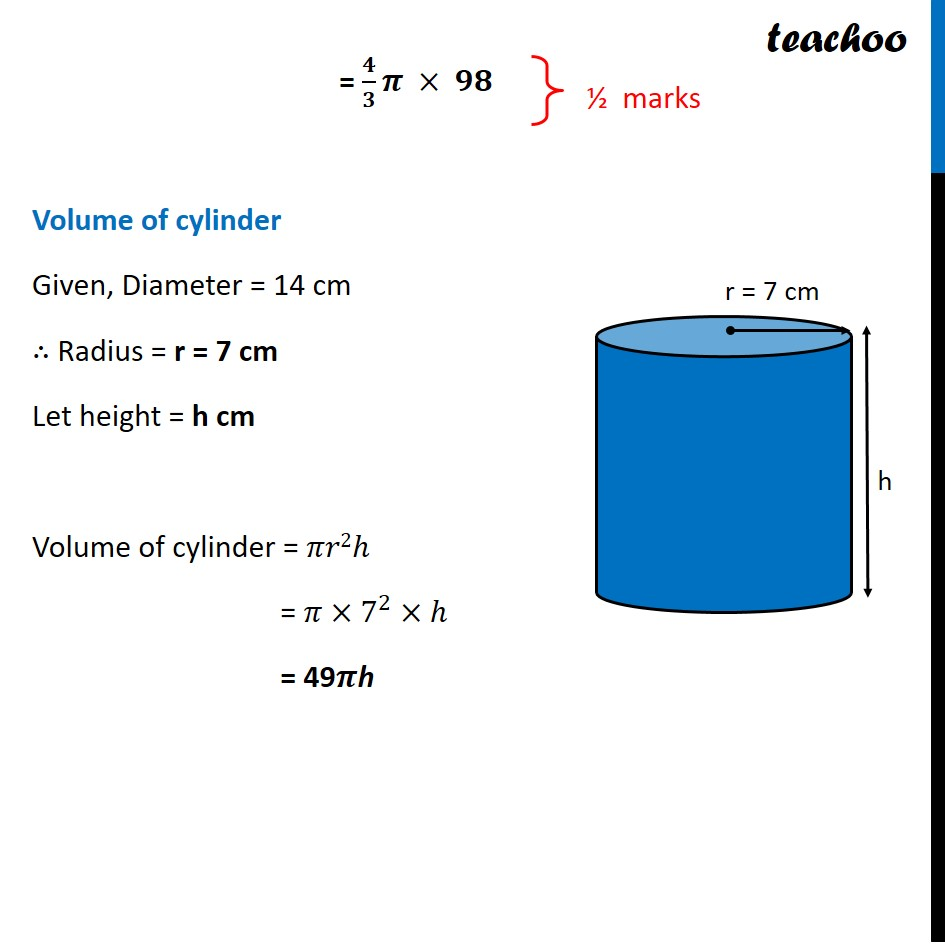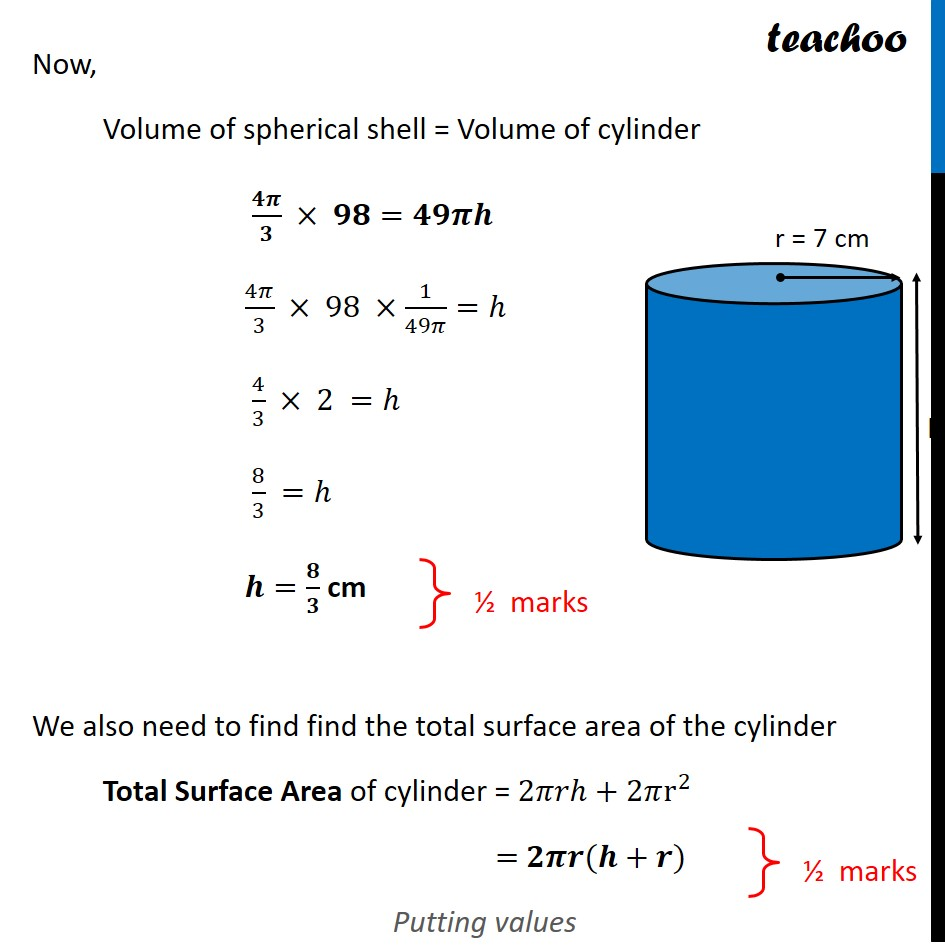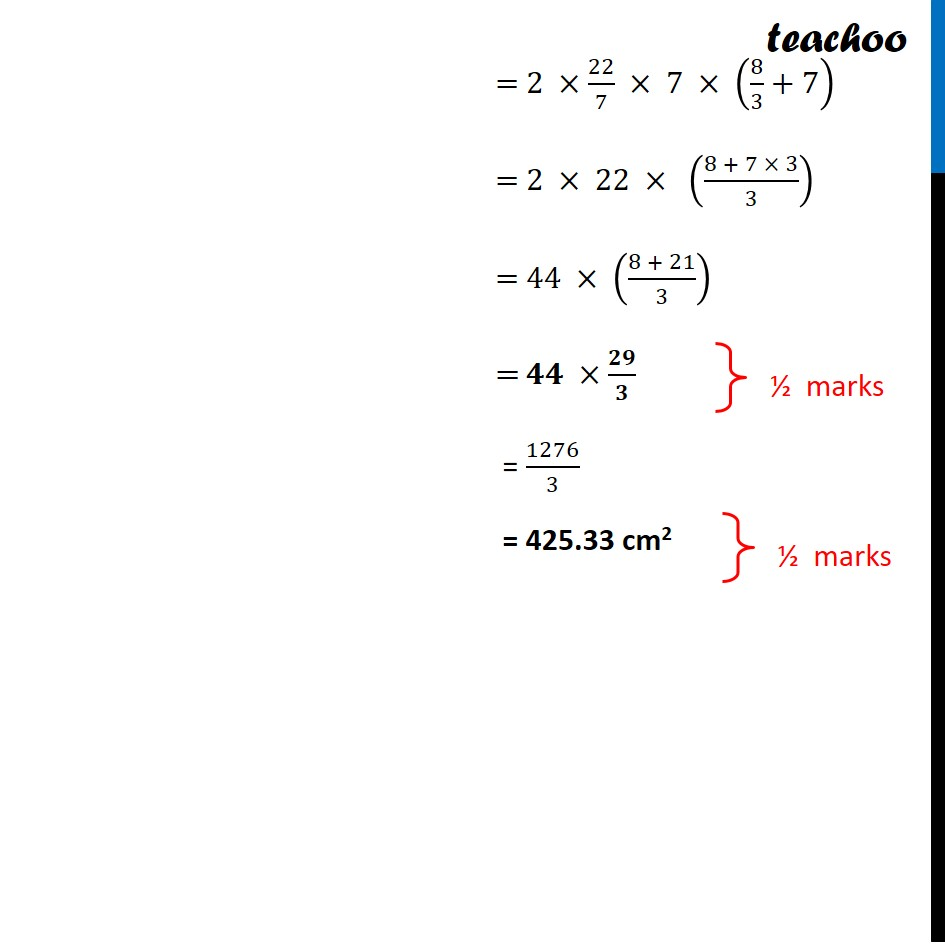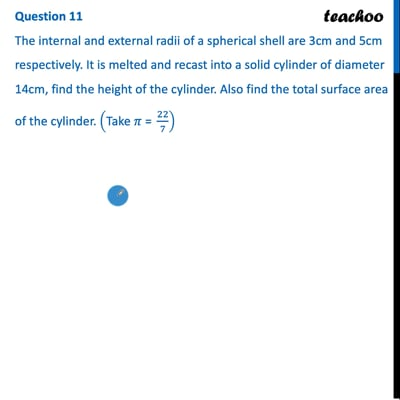This video is only available for Teachoo black users

### Transcript

The internal and external radii of a spherical shell are 3cm and 5cm respectively. It is melted and recast into a solid cylinder of diameter 14cm, find the height of the cylinder. Also find the total surface area of the cylinder. ("Take 𝜋 = " 22/7) Since spherical shell is melted into a cylinder , So, Volume of spherical shell = Volume of cylinder Volume of spherical shell Internal Radius = r = 3 cm External Radius = R = 5 cm Volume of spherical shell = 4/3 𝜋𝑅3−4/3 𝜋𝑟3 = 𝟒/𝟑 𝝅(𝑹^𝟑−𝒓^𝟑) = 4/3 𝜋(5^3−3^3) = 4/3 𝜋(126−27) = 𝟒/𝟑 𝝅 × 𝟗𝟖 Volume of cylinder Given, Diameter = 14 cm ∴ Radius = r = 7 cm Let height = h cm Volume of cylinder = 𝜋𝑟2ℎ = 𝜋×7^2×ℎ = 49𝝅h Now, Volume of spherical shell = Volume of cylinder 𝟒𝝅/𝟑 × 𝟗𝟖=𝟒𝟗𝝅𝒉 4𝜋/3 × 98 ×1/49𝜋=ℎ 4/3 × 2 =ℎ 8/3 =ℎ 𝒉=𝟖/𝟑 cm We also need to find find the total surface area of the cylinder Total Surface Area of cylinder = 2𝜋𝑟ℎ+2𝜋r^2 = 𝟐𝝅𝒓(𝒉+𝒓) Putting values = 2 ×22/7 × 7 × (8/3+7) = 2 × 22 × ((8 + 7 × 3)/3) = 44 × ((8 + 21)/3) = 𝟒𝟒 ×𝟐𝟗/𝟑 = 1276/3 = 425.33 cm2